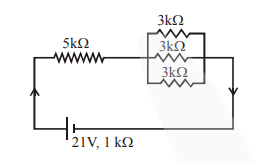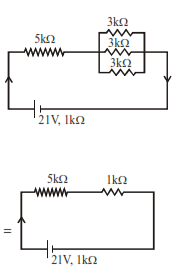# In the figure given, the electric current flowingQuestion:

In the figure given, the electric current flowing through the $5 \mathrm{k} \Omega$ resistor is ' $\mathrm{x}$ ' $\mathrm{mA}$.The value of x to the nearest integer is_________

Solution:$I=\frac{21}{5+1+1}=3 \mathrm{~mA}$